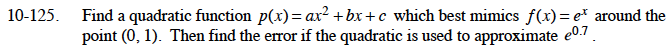Home > CALC > Chapter Ch10 > Lesson 10.3.2 > Problem10-125

10-125.

Find a quadratic function p(x) = ax2 + bx + c which best mimics f(x) = ex around the point (0, 1). Then find the error if the quadratic is used to approximate e0.7. Homework Help ✎Error: p(0.7) – f(0.7)